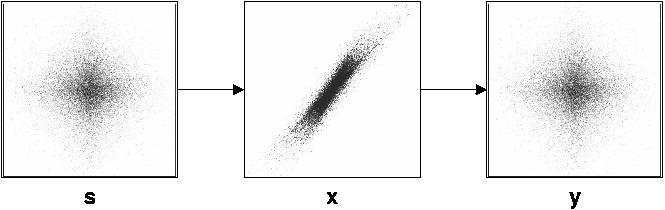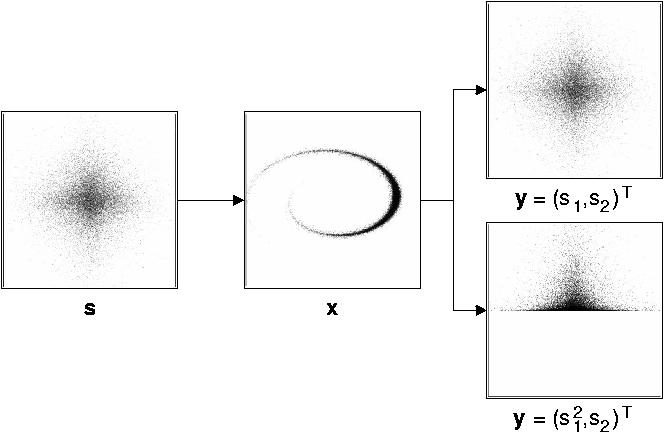• RUB
• INI
• Projects
• Independent slow feature analysis and nonlinear blind source separation
Independent slow feature analysis and nonlinear blind source separationCollaborator: Tobias Blaschke, Tiziano Zito

If two statistically independent sources are mixed linearly, then the original source signals can be recovered from the mixture simply by finding a linear transformation that generates statistically independent components. This can be performed by standard linear ICA, which in this case is equivalent to linear blind source-separation. An example is given in Figure 1.Figure 1: Example of linear mixing and unmixing of two sources. In this case there is basically only one way to get statistically independent signal components from the observed signal x (except for permutations and scaling).

In the nonlinear case the situation is more complicated. If thuuuuiiiFigure 2: Example of nonlinear mixing and unmixing of two sources. In this case there are many ways of extracting two statistically independent signal components from the observed signal; only two ways are shown here. (The nonlinear mixture has been taken from a paper by Harmeling et al. (2003))

To solve this problem of nonlinear blind source separation one needs an additional criterion to select the correct solution from all the other ones. Here helps the following observation. Given any signal with some temporal continuity, a nonlinear non-invertible transformation of that signal will typically produce a signal that is more quickly varying than the original one. Squaring a sine wave, for instance, leads to frequency doubling and therefore to a faster signal. Thus, we can use the temporal-slowness objective to select the original sources.

In this project we have integrated ICA and SFA into an algorithm that we call Independent Slow Feature Analysis (ISFA), which is able to perform nonlinear blind source separation as indicated in the top right example of Figure 2 (original results shown in the papers).